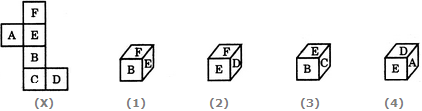# Non Verbal Reasoning - Cubes and Dice - Discussion

### Discussion :: Cubes and Dice - Section 1 (Q.No.7)

The sheet of paper shown in the figure (X) given on the left hand side, in each problem, is folded to form a box. Choose from amongst the alternatives (1), (2), (3) and (4), the boxes that are similar to the box that will be formed.

7.

Choose the box that is similar to the box formed from the given sheet of paper (X).[A]. 1 only [B]. 2 only [C]. 1 and 3 only [D]. 1, 2, 3 and 4 only

Answer: Option B

Explanation:

The fig. (X) is similar to the Form III. So, when the sheet in fig. (X) is folded to form a cube, then 'F' appears opposite 'B', 'E' appears opposite 'C' and 'A' appears opposite 'D' Therefore, the cube in fig. (1) which shows 'F' adjacent to 'B' the cube in fig. (3) which shows 'E' adjacent to 'C' and the cube in fig. (4) which shows 'A' adjacent to 'D' cannot be formed. Hence, only the cube in fig.(2) can be formed.

 Bla Hi said: (Feb 1, 2015) Shouldn't the faces be rotated (e.g. A is sideways)?

 Deen said: (Jun 24, 2016) Simply make the diagram (cube) first then see the possible figures as per the given statement.

 Pankaj Verma said: (Nov 22, 2017) Can anyone kindly explain the diagram?

 Biswajit Das said: (May 15, 2018) Thanks for explaining.

 Sankar said: (Sep 22, 2018) D is always right side of FEBC and A is always left side of this.

 Rupankar said: (Oct 21, 2018) I am not getting this problem. Please explain me.

 Shewaram said: (Nov 5, 2018) Opposite sides are never together here F to B and E to C and A to D. In first F and B are together and in third E and C are together in fourth there is A and D are together therefore 2 is the answer.

 Durga Bhavani said: (Jan 11, 2019) Here E opposite to C; B opposite to F; A opposite to D. By this you will get the answer.

 Padmasri said: (Apr 20, 2019) Thanks for explaining.

 Lal said: (Sep 26, 2019) Good, Thanks all for explaining.

#### Post your comments here:

Name *:

Email   : (optional)

» Your comments will be displayed only after manual approval.

#### Current Affairs 2021

Interview Questions and Answers